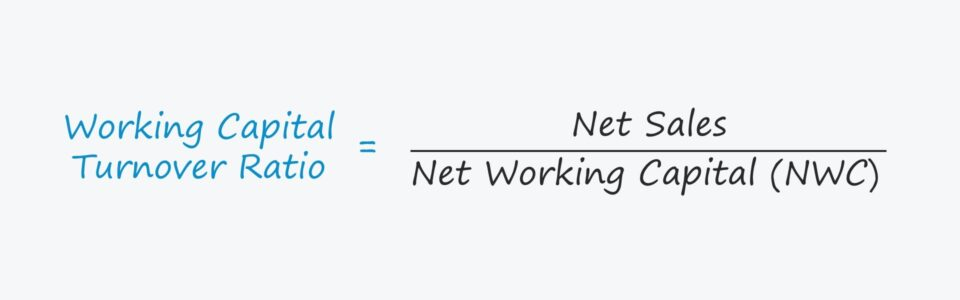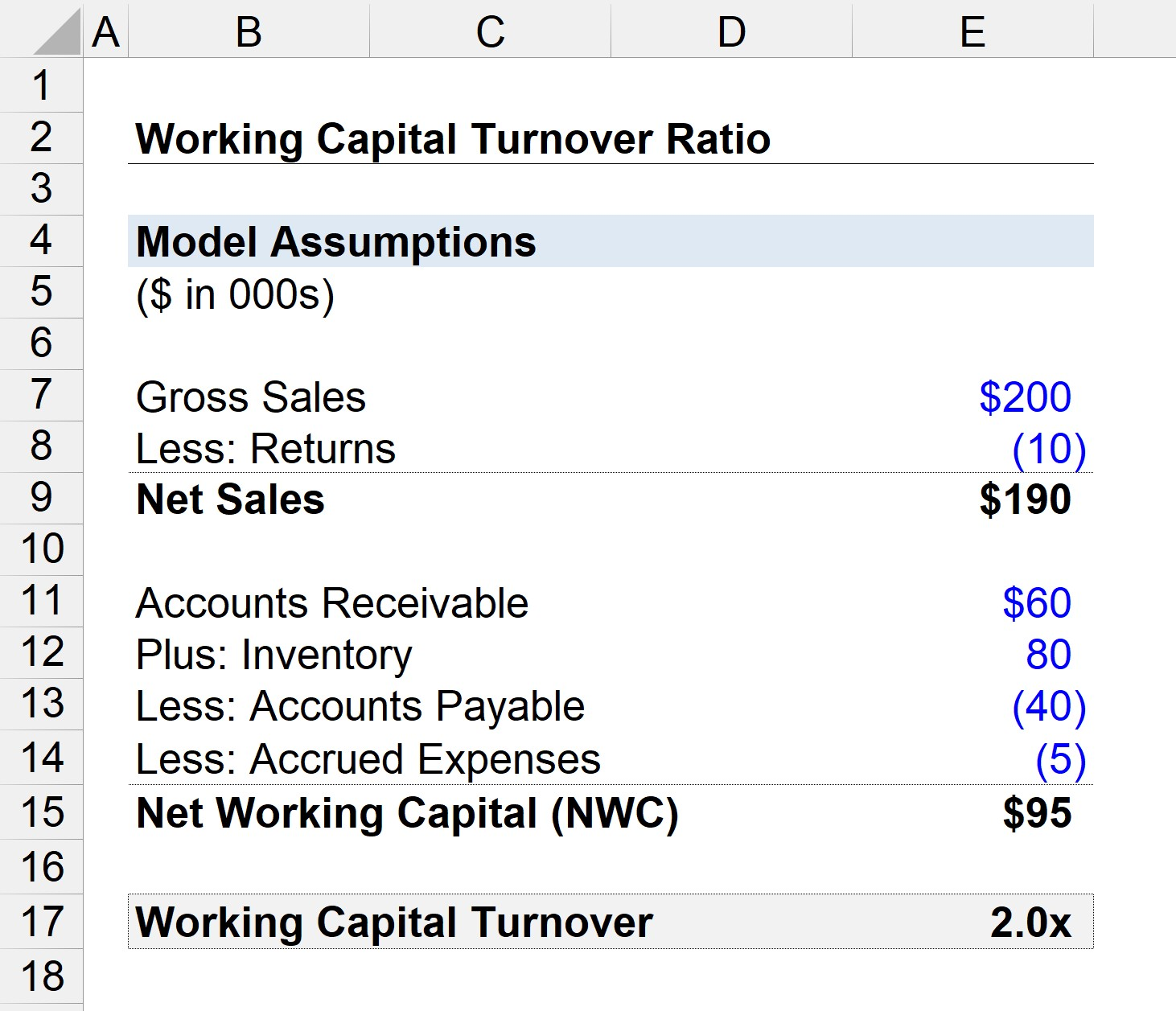# Working Capital Turnover

Understand the Working Capital Turnover Concept• What is the definition of the working capital turnover ratio?
• What does the working capital turnover ratio measure?
• What formula calculates the working capital turnover ratio?
• Is a higher or lower working capital turnover ratio preferred?

## Working Capital Turnover Formula

The working capital turnover compares a company’s net sales to its net working capital (NWC) in an effort to gauge its operating efficiency.

The NWC turnover metric can be a useful tool for evaluating how efficiently a company is utilizing its working capital to produce more revenue.

The formula for calculating the net working capital (NWC) divides a company’s net sales (i.e. turnover) by its net working capital (NWC).

### NWC Turnover Ratio Formula

• Working Capital Turnover = Net Sales / Net Working Capital (NWC)

The sales of a business are reported on its income statement, which tracks activity over a period of time.

In order to match the time period of the numerator with that of the denominator, using the average NWC balances between the beginning and ending periods is recommended.

However, unless the company’s NWC has changed drastically over time, the difference between using the average NWC value compared to the ending balance value is rarely significant.

While the working capital metric can be used – i.e. current assets minus current liabilities – the net working capital (NWC) is a more practical measure since only operating assets and liabilities are included.

• Non-operating assets such as cash and cash equivalents like marketable securities are excluded in the calculation of net working capital (NWC).
• Non-operating liabilities like debt and other interest-bearing securities are also removed because they represent financial liabilities.

## Interpreting the Working Capital Turnover Ratio

The working capital turnover can be interpreted as the amount of sales created for each dollar of working capital owned.

• High Turnover → Since a higher working capital turnover ratio implies the company’s working capital management is more efficient, most companies aim to increase the number of “turns.”
• Low Turnover → On the other hand, a lower working capital turnover ratio would suggest the opposite, i.e. the company’s working capital spending and day-to-day management are inefficient.

If a company’s turnover ratio is trailing behind its peers, this may be a sign it may need to further optimize its operational practices, as its sales are insufficient compared to the amount of working capital put to use.

For instance, a NWC turnover ratio of 3x indicates that the company generates \$3 of sales per dollar of working capital employed.

The turnover ratio portrays the efficiency at which a company’s operations can create sales, which supports the statement from earlier about net working capital (NWC) being preferable over working capital.

In order words, assets such as cash and liabilities such as debt are financial assets that are not necessarily tied to the core operations of a company.

In particular, comparisons among different companies can be less meaningful if the effects of discretionary financing choices by management are included.

## Working Capital Turnover Ratio Calculator

We’ll now move to a modeling exercise, which you can access by filling out the form below.Submitting ...

## Working Capital Turnover Ratio Example Calculation

Suppose a business had \$200,000 in gross sales in the past year, with \$10,000 in returns.

• Net Sales = \$200,000 – \$10,000 = \$190,000

The average balances of the company’s net working capital (NWC) line items – i.e. calculated as the sum of the ending and beginning balance divided by two – are shown below.

Using the assumptions above, the net working capital (NWC) equals the difference between operating current assets minus operating current liabilities, which comes out to be \$95,000.

• Net Working Capital (NWC) = (\$60,000 + \$80,000) – (\$40,000 + \$5,000)
• NWC = \$95,000

Since we now have the two necessary inputs to calculate the working capital turnover, the remaining step is to divide net sales by NWC.

• Working Capital Turnover = \$190,000 / \$95,000 = 2.0x

From the 2.0x working capital turnover ratio, we can conclude that the business generates \$2 in net sales for each dollar of net working capital (NWC) employed.Step-by-Step Online Course

#### Everything You Need To Master Financial Modeling

Enroll in The Premium Package: Learn Financial Statement Modeling, DCF, M&A, LBO and Comps. The same training program used at top investment banks.Inline FeedbacksLearn Financial Modeling Online

Everything you need to master financial and valuation modeling: 3-Statement Modeling, DCF, Comps, M&A and LBO.

X

The Wall Street Prep Quicklesson Series

7 Free Financial Modeling Lessons

Get instant access to video lessons taught by experienced investment bankers. Learn financial statement modeling, DCF, M&A, LBO, Comps and Excel shortcuts.